Courses

# Effective Stress, Capillarity & Permeability of Soils Notes | EduRev

## Civil Engineering (CE) : Effective Stress, Capillarity & Permeability of Soils Notes | EduRev

The document Effective Stress, Capillarity & Permeability of Soils Notes | EduRev is a part of the Civil Engineering (CE) Course Soil Mechanics.
All you need of Civil Engineering (CE) at this link: Civil Engineering (CE)

Well Hydraulics

1. Specific yield (Sy)
The specific yield of an unconfined aquifer is the ratio of volume of water which will flow under saturated condition due to gravity effect to the total volume of aquifer (v).
Sy = Vwy/V where, Vwy = Volume of water yielded under gravity effect and V = total volume of water.
2. Specific retention: The specific retention of an unconfined aquifer is the ratio of volume of water retained against gravity effect to the total volume of aquifer (v).
SR = VWR/V where, VWR = Volume of water retained under gravity effect.

Coefficient of transmissibility
T = kH where, H = Thickness
k = Coefficient of permeability

Unconfined Aquifer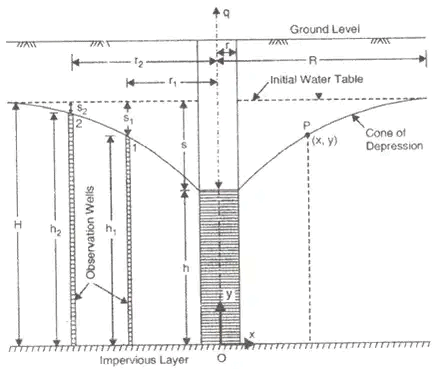1. Theims Theory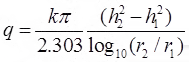h1 + s1 = h2 + s2
where, q = Rate of flow in m3/s
h= Height of water table of 1st observation well
h2 = Height of water table of 2nd observation well
s1 = Drawdown of 1st test well
s2 = Drawdown of 2nd test well.
r1 = and r2 are radius of 1st and 2nd observation wells respectively.
2. Dupits Theory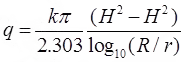R = 3000.S√K and S = H - h
Where, S = Drawdown in the well
k = Permeability coefficient in m/s.
R = Radius of influence in 'm'
150m ≤ R ≤ 300m
r = Radius of tes well in 'm'.
Results of dupits theory are not accurate because 'R' is based on empirical relation.

Confined Aquifer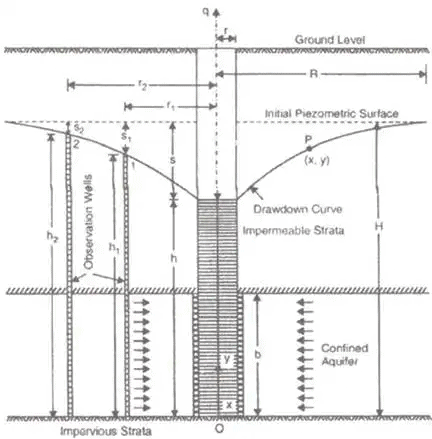1. Theims theorywhere, b = width
2. Dupits theory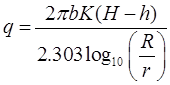Spherical flow through well
qs = K.2πr.s where, r = Radius of well
S = Drawdown
qs = Rate of flow through spherical well in m3/s q= 1/30.qradial flow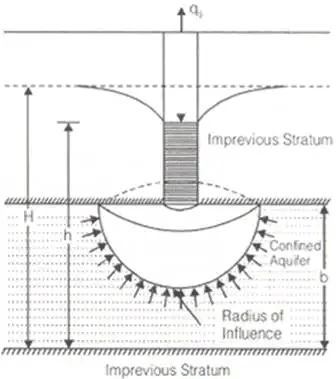Pumping-In-Test

1. Open end test
K = q/5.5rh where, r = Radius of pipe
h = Head of water above the base of pipe, it may include gravity head and pressure head.
2. Tacker test
K = q/(2πLh)log10(L/r) ... when L > 10r
where, L = Length of perforated section of pipe
k = q/(2πLh)sin - 1(L/2r) ... when L < 10 r

Open well (Recuperation test)
q = (C/A).Volume
Where, C/A = (2.303/T)log10(h1/h2)
C/A = Specific yield or specific capacity of an open well.
T = Time in 'sec'
h1 = Position of water table of t = 0
h2 = Position of water table of t = T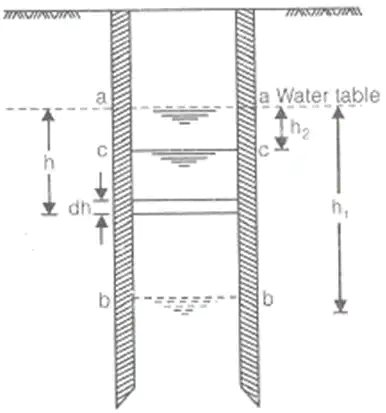Value of Permeability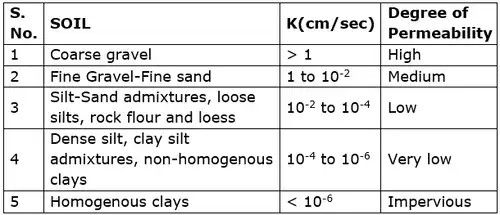Offer running on EduRev: Apply code STAYHOME200 to get INR 200 off on our premium plan EduRev Infinity!

## Soil Mechanics

31 videos|48 docs|42 tests

,

,

,

,

,

,

,

,

,

,

,

,

,

,

,

,

,

,

,

,

,

,

,

,

;